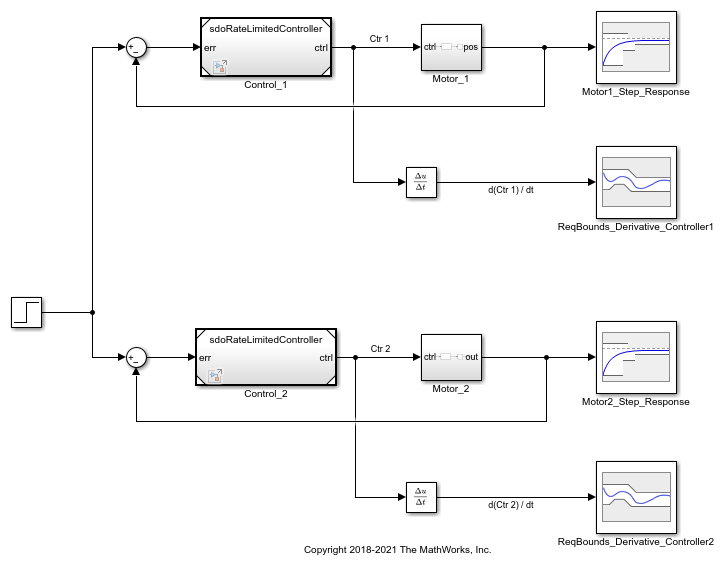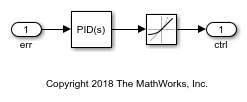# Design Optimization Tuning Parameters in Referenced Models (Code)

This example shows how to tune parameters in referenced models, using the `sdo.optimize` command.

### Motor Control Model

The model shows the control of angular position for two motors. Open the Simulink model.

```open_system('sdoMultipleMotors') ```Two motors are controlled. Model references are used for the controllers, which are instances of the same model. Open the controller.

```open_system('sdoRateLimitedController') ```### Design Problem

The two motors in the main model have different characteristics, so each controller needs to be tailored to its motor. Each controller has PID gains `Kp`, `Ki`, and `Kd`, and a slew rate, `Slew`. The `Slew` value limits the rate at which the control signal changes. The `Slew` value is common to both instances of the controller. In contrast, the PID gains need to be different for each instance of the controller, since the motors being controlled have different characteristics. Therefore, the PID gains are specified as model arguments in the controller's model workspace. The PID gain values are set at the level of the `sdoMultipleMotors` model.

The control reference signal is a step change in position, which occurs at 1 second. Each motor's angular position should follow the reference signal, but the first motor has a smaller moment of inertia and can respond more quickly to changes in the reference signal. Also, the derivative of each controller signal must be limited, so that currents drawn from the power supply stay within the power supply's limits.

### Specify Design Variables

Specify the design variables to be tuned by the optimization routine in order to satisfy the requirements. The `Slew` variable is specified as `sdoRateLimitedController:Slew`, indicating that the variable is set at the level of the `sdoRateLimitedController` model. The colon is a delimiter between the model and variable. The `Slew` variable has the same value for all instances of the controller model. In contrast, the proportional gain for the first controller is specified as `sdoMultipleMotors/Control_1:Kp`, indicating that the variable is set at the level of the `sdoMultipleMotors` model, where it appears in the `Control_1` block. The forward slash is the delimiter for Simulink blockpaths.

Specify that design variables include the gains `Kp`, `Ki`, and `Kd`, for both PID controllers. Also include the slew rate, `Slew`, which is common to both controllers. Finally, specify that the slew rate cannot be negative.

```DesignVars = sdo.getParameterFromModel('sdoMultipleMotors', ... {'sdoRateLimitedController:Slew', ... ... 'sdoMultipleMotors/Control_1:Kp', ... 'sdoMultipleMotors/Control_1:Ki', ... 'sdoMultipleMotors/Control_1:Kd', ... ... 'sdoMultipleMotors/Control_2:Kp', ... 'sdoMultipleMotors/Control_2:Ki', ... 'sdoMultipleMotors/Control_2:Kd' }); DesignVars(1).Minimum = 0; ```

### Specify Design Requirements

Each motor's angular position angle should follow the reference signal, but the first motor has a smaller moment of inertia and can respond more quickly to changes in the reference signal. We want the angular position of the first motor to satisfy the following requirements:

• Rise time: 2 seconds

• Settling time: 7 seconds

This requirement is specified in a step response check block in the Simulink model. We can refer to the block and include the requirement in a variable, to be passed to the optimization objective function.

```Requirements = struct; bnds = getbounds('sdoMultipleMotors/Motor1_Step_Response'); Requirements.Motor1_StepResponse = bnds{1}; ```

The second motor has a larger moment of inertia, so it can't respond as quickly. We want the angular position of the second motor to satisfy the following requirements:

• Rise time: 8 seconds

• Settling time: 10 seconds

This requirement is also specified in a step response check block in the Simulink model, and we refer to the block to include the requirement in a variable, to be passed to the optimization objective function.

```bnds = getbounds('sdoMultipleMotors/Motor2_Step_Response'); Requirements.Motor2_StepResponse = bnds{1}; ```

Also, the derivative of each controller signal is required to be in the range from -5 to 5, so that currents drawn from the power supply stay within the power supply's limits. These requirements are specified in bound check blocks in the Simulink model, and these requirements should also be collected among requirements to be passed to the optimization objective function.

```bnds = getbounds('sdoMultipleMotors/ReqBounds_Derivative_Controller1'); Requirements.Controller1_DerivBound1 = bnds{1}; Requirements.Controller1_DerivBound2 = bnds{2}; bnds = getbounds('sdoMultipleMotors/ReqBounds_Derivative_Controller2'); Requirements.Controller2_DerivBound1 = bnds{1}; Requirements.Controller2_DerivBound2 = bnds{2}; ```

Prevent check block assertions during optimization.

```CheckBlockStatus = sdo.setCheckBlockEnabled('sdoMultipleMotors','off'); ```

### Simulation Definition

The cost function requires a simulation scenario to run the model. Create a simulation scenario and add model signals to log, so their values are available to the cost function.

```Simulator = sdo.SimulationTest('sdoMultipleMotors'); ```

The motor angular positions need to be logged during optimization, to evaluate the requirements on their step responses.

```Motor1_Position = Simulink.SimulationData.SignalLoggingInfo; Motor1_Position.BlockPath = 'sdoMultipleMotors/Motor1_Step_Response/u'; Motor1_Position.LoggingInfo.LoggingName = 'Motor1_Position'; Motor1_Position.LoggingInfo.NameMode = 1; Motor2_Position = Simulink.SimulationData.SignalLoggingInfo; Motor2_Position.BlockPath = 'sdoMultipleMotors/Motor2_Step_Response/u'; Motor2_Position.LoggingInfo.LoggingName = 'Motor2_Position'; Motor2_Position.LoggingInfo.NameMode = 1; ```

The controller signal derivatives also need to be logged, to evaluate the bound requirements on them.

```Controller1_Derivative = Simulink.SimulationData.SignalLoggingInfo; Controller1_Derivative.BlockPath = 'sdoMultipleMotors/ReqBounds_Derivative_Controller1/u'; Controller1_Derivative.LoggingInfo.LoggingName = 'Controller1_Derivative'; Controller1_Derivative.LoggingInfo.NameMode = 1; Controller2_Derivative = Simulink.SimulationData.SignalLoggingInfo; Controller2_Derivative.BlockPath = 'sdoMultipleMotors/ReqBounds_Derivative_Controller2/u'; Controller2_Derivative.LoggingInfo.LoggingName = 'Controller2_Derivative'; Controller2_Derivative.LoggingInfo.NameMode = 1; ```

To log these signals during optimization, collect them into the simulation scenario, `Simulator`.

```Simulator.LoggingInfo.Signals = [... Motor1_Position ; ... Motor2_Position ; ... Controller1_Derivative ; ... Controller2_Derivative ]; ```

### Create Optimization Objective Function

Create an objective function, which will be called at each optimization iteration, to evaluate the design requirements as the design variables are tuned. This cost function has input arguments for the design variables, simulation scenario, and design requirements.

```type sdoMultipleMotors_Design ```
```function Vals = sdoMultipleMotors_Design(P,Simulator,Requirements) %SDOMULTIPLEMOTORS_DESIGN Objective function for multiple motors % % Function called at each iteration of the optimization problem. % % The function is called with the model named mdl, a set of parameter % values, P, a Simulator, and the design Requirements to evaluate. It % returns the objective value and constraint violations, Vals, to the % optimization solver. % % See the sdoExampleCostFunction function and sdo.optimize for a more % detailed description of the function signature. % % See also sdoMultipleMotors_cmddemo % Copyright 2018 The MathWorks, Inc. %% Model Evaluation % Simulate the model. Simulator.Parameters = P; Simulator = sim(Simulator); % Retrieve logged signal data. SimLog = find(Simulator.LoggedData, get_param('sdoMultipleMotors','SignalLoggingName')); Motor1_Position = find(SimLog,'Motor1_Position'); Motor2_Position = find(SimLog,'Motor2_Position'); Controller1_Derivative = find(SimLog,'Controller1_Derivative'); Controller2_Derivative = find(SimLog,'Controller2_Derivative'); % Evaluate the design requirements. Cleq_Motor1_StepResponse = evalRequirement(Requirements.Motor1_StepResponse, Motor1_Position.Values); Cleq_Motor2_StepResponse = evalRequirement(Requirements.Motor2_StepResponse, Motor2_Position.Values); Cleq_Controller1_DerivBound1 = evalRequirement(Requirements.Controller1_DerivBound1, Controller1_Derivative.Values); Cleq_Controller1_DerivBound2 = evalRequirement(Requirements.Controller1_DerivBound2, Controller1_Derivative.Values); Cleq_Controller2_DerivBound1 = evalRequirement(Requirements.Controller2_DerivBound1, Controller2_Derivative.Values); Cleq_Controller2_DerivBound2 = evalRequirement(Requirements.Controller2_DerivBound2, Controller2_Derivative.Values); %% Return Values. % % Collect the evaluated design requirement values in a structure to % return to the optimization solver. Vals.Cleq = [... Cleq_Motor1_StepResponse(:); ... Cleq_Motor2_StepResponse(:); ... Cleq_Controller1_DerivBound1(:); ... Cleq_Controller1_DerivBound2(:); ... Cleq_Controller2_DerivBound1(:); ... Cleq_Controller2_DerivBound2(:)]; end ```

To optimize, define a handle to the cost function that uses the `Simulator` and `Requirements` defined above. Use an anonymous function that takes one argument (the design variables) and calls the objective function. Finally, call sdo.optimize to optimize the design variables to try to meet the requirements.

```optimfcn = @(P) sdoMultipleMotors_Design(P, Simulator, Requirements); [Optimized_DesignVars, Info] = sdo.optimize(optimfcn, DesignVars); ```
``` Optimization started 01-Sep-2021 16:52:24 max First-order Iter F-count f(x) constraint Step-size optimality 0 15 0 211.8 1 30 0 8.464 2.92 26.5 2 50 0 6.531 0.396 15.1 3 70 0 1.931 0.675 15.7 4 85 0 0.242 1.67 41.2 5 100 0 0.04523 0.272 1.02e+03 6 110 0 0.04523 0.00398 0 Local minimum found that satisfies the constraints. Optimization completed because the objective function is non-decreasing in feasible directions, to within the value of the optimality tolerance, and constraints are satisfied to within the value of the constraint tolerance. ```

Restore check block assertions.

```sdo.setCheckBlockEnabled('sdoMultipleMotors', CheckBlockStatus); ```

### Update Model

Update the model with the optimized parameter values.

```sdo.setValueInModel('sdoMultipleMotors',Optimized_DesignVars); ```

Close the models.

```bdclose('sdoMultipleMotors') bdclose('sdoRateLimitedController') ```

## Related Topics